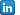February 2019

by @mw

## Testing conditional expectations

I recently came across a problem of testing if the expectations of one variable, call it \$Y\$, vary alongside the distribution of another variable, say \$X\$. The problem can be approached through several angles, including parametric quantile approach, however, it was decided to use one of the most flexible methods, and actually one of my favorites, i.e. the bootstrap.

The idea is quite simple. Imagine two random variables \$Y\$ and \$X\$. (For more information about the exact definitions of what a random variable is, the Wikipedia page has a lot of useful information.) Given their observed realisations \$\{(Y_i,X_i):i=1,...,n\}\$, the goal is to test if the conditional average of \$Y\$ is statistically different from its unconditional average. We can approximate the former by estimating the mean of \$Y\$ for different parts of \$X\$ distribution. For instance, we can test if expectations of \$Y\$ differ if \$X\$ falls below different percentiles of its distribution. Of course, it is not the only possible strategy, but lets stick to it for the moment, for the illustration.

I construct the test to verify if the conditional expectations are statistically different from the unconditional one, or in other words, if the mean of \$Y\$ in certain areas is statistically different from the overall mean. Such a setup can be useful, for instance, to check if the relationship between the variables is of U-shape (to do that one would need to evaluate the test for two tails of \$X\$). Formally, given q-percentile of \$X\$, \$x_q\$ where \$q ∈ (0,1)\$, under the null hypothesis the conditional and unconditional expectations are equal, i.e.

\$\$ H_0: E[Y|X ≤x_q] = E[Y]. \$\$

To find out if the data contain sufficient evidence against the null hypothesis, we need somehow to measure the information contained by the data, i.e. to find the test statistic. In our setup the base value of interest is the expectation of \$Y\$ so that the test statistic is the conditional expectation. If we denote the number of times \$X\$ falls below \$x_q\$ by \$n_1\$, the conditional expectation estimator is given by

\$\$ T* = E[Y|X ≤x_q] = {1}/{n_1} ∑↙{i=0}↖{n} Y_i I_{X_i≤x_q} , \$\$

where \$I\$ is an indicator function taking value \$1\$ if condition is met and \$0\$ otherwise. Given that \$T*\$ captures the evidence against the equivalence with unconditional expectations given the observed data, the last thing we need to assess its statistical significance is to approximate what would have been its distribution if the equivalence condition is met. This is where the bootstrap comes handy.

In a nutshell, the idea is to impose the condition from the null hypothesis onto the data by permuting or reshuffling the observed realisations. The difference between the two is that whereas the former changes the order of original data, the latter does allow for replacement sampling. In our example we want to break the link between the conditional and unconditional expectations, which we observe in the data. We could achieve it by randomly re-assigning for each realisation of \$X=X_i\$ a new realisation of \$Y\$ from an observed set of \$\{Y_i:i=1,...,n\}\$. In such a way, we mimic the situation that samples \$\{X_i\}\$ and \$\{Y\}\$ were drawn independently, so the relation between them across any point in the distribution should be nothing more than random.

To get the statistical significance for the test we can apply a simple procedure proposed by Efron and Tibshirani (1993) [see page 221]. Draw \$B\$ bootstrap samples from \$Y\$ with replacement. Evaluate the test statistic for each bootstrap sample \$b=1,...,B\$ and call it \$T^b\$. Statistical significance of \$T^*\$ can be approximated as

\$\$ p = {#\{ T^b ≥ T^* \}} / B \$\$

A simple code snippet to apply the above logic in practice is given below. It uses the

`boot`
package.
```#define the test statistic function (this is needed for the boot package)
stat = function(d, ids, q = 0.1, tail = "lower") {

xd <- d[,1]
yd <- d[ids,2]

if(tail == "lower") return(mean(yd[xd <= quantile(xd, probs = q)]))
if(tail == "upper") return(mean(yd[xd >= quantile(xd, probs = 1-q)]))
}

#setup (sample size is determined by n)
n <- 100
x <- rnorm(mean = 0, sd = 1, n)
y <- rnorm(mean = x^2, sd = 1, n)

#bootstrap
require(boot)
B0   <- boot(cbind(x,y), stat, R = 999, q=0.1, tail = "upper")
pVal <- mean(B0\\$t > B0\\$t0)
plot(B0)
```

In the example above the \$Y\$ variable is generated from a quadratic relation against \$X\$ so that the null hypothesis is violated. As expected, the test delivers low p values. On the other hand, if you replace the \$Y\$ as a standard normal variable, i.e. independent from \$X\$, the effect disappear.

References

Efron, B. and Tibshirani, R.J. (1993) An Introduction to the Bootstrap. Chapman & Hall, New York.Marcin Wolski, PhDView my profileIDEAS/RePEc